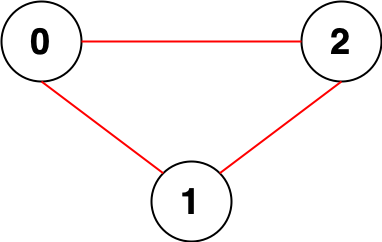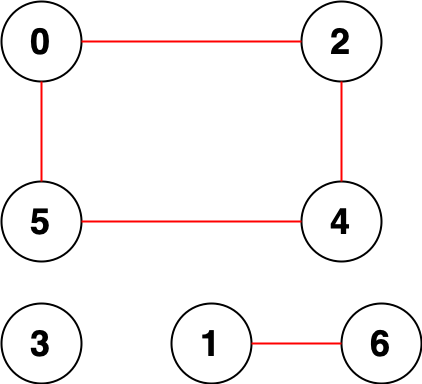# 2316. Count Unreachable Pairs of Nodes in an Undirected Graph

Medium
You are given an integer `n`. There is an undirected graph with `n` nodes, numbered from `0` to `n - 1`. You are given a 2D integer array `edges` where `edges[i] = [ai, bi]` denotes that there exists an undirected edge connecting nodes `ai` and `bi`.
Return the number of pairs of different nodes that are unreachable from each other.
Example 1:Input: n = 3, edges = [[0,1],[0,2],[1,2]]
Output: 0
Explanation: There are no pairs of nodes that are unreachable from each other. Therefore, we return 0.
Example 2:Input: n = 7, edges = [[0,2],[0,5],[2,4],[1,6],[5,4]]
Output: 14
Explanation: There are 14 pairs of nodes that are unreachable from each other:
[[0,1],[0,3],[0,6],[1,2],[1,3],[1,4],[1,5],[2,3],[2,6],[3,4],[3,5],[3,6],[4,6],[5,6]].
Therefore, we return 14.
Constraints:
• `1 <= n <= 10^5`
• `0 <= edges.length <= 2 * 10^5`
• `edges[i].length == 2`
• `0 <= ai, bi < n`
• `ai != bi`
• There are no repeated edges.

### 解題

func countPairs(n int, edges [][]int) int64 {
root := make([]int, n)
size := make([]int, n)
visited := make([]bool, n)
for i:=0; i<n; i++ {
root[i] = i
size[i] = 1
}
for _, edge := range edges {
combine(root, edge, edge, size)
}
ans := 0
for i:=0; i<n; i++ {
f := find(root, i)
if visited[f] { continue }
visited[f] = true
ans += size[f]*(n-size[f])
}
return int64(ans/2)
}
func combine(root []int, a int, b int, size []int) {
a = find(root, a)
b = find(root, b)
if a==b { return }
root[b] = a;
size[a] += size[b]
}
func find(root []int, x int) int {
if root[x] == x { return x }
return find(root, root[x])
}